Next: What is the Delaunay Up: Voronoi Diagram and Delaunay Previous: What is cell complex?   Contents

## What is Voronoi diagram in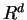?

See also 3.3.

Given a set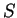of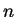distinct points in, Voronoi diagram is the partition ofintopolyhedral regions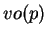(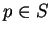). Each region, called the Voronoi cell of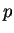, is defined as the set of points inwhich are closer tothan to any other points in, or more precisely,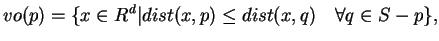where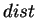is the Euclidean distance function. (One can use different distance functions to define various variations of Voronoi diagrams, but we do not discuss them here.)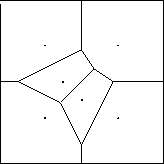The set of all Voronoi cells and their faces forms a cell complex. The vertices of this complex are called the Voronoi vertices, and the extreme rays (i.e. unbounded edges) are the Voronoi rays. For each point, the nearest neighbor set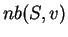of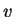inis the set of pointswhich are closest toin Euclidean distance. Alternatively, one can define a pointto be a Voronoi vertex ofifis maximal over all nearest neighbor sets.

In order to compute the Voronoi diagram, the following construction is very important. For each pointin, consider the hyperplane tangent to the paraboloid in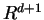at: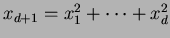. This hyperplane is represented by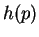:By replacing the equality with inequality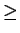above for each point, we obtain the system ofinequalities, which we denote by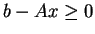. The polyhedron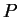inof all solutions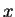to the system of inequalities is a lifting of the Voronoi diagram to one higher dimensional space. In other words, by projecting the polyhedrononto the originalspace, we obtain the Voronoi diagram in the sense that the projection of each facet ofassociated withis exactly the voronoi cell. The vertices and the extreme rays ofproject exactly to the Voronoi vertices and the rays, respectively.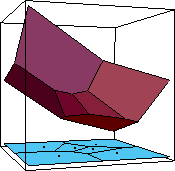Next: What is the Delaunay Up: Voronoi Diagram and Delaunay Previous: What is cell complex?   Contents
Komei Fukuda 2004-08-26